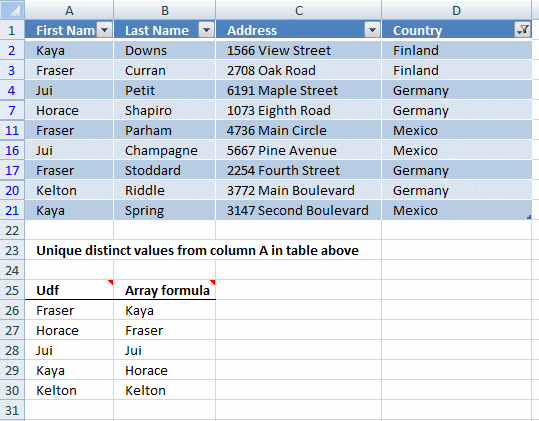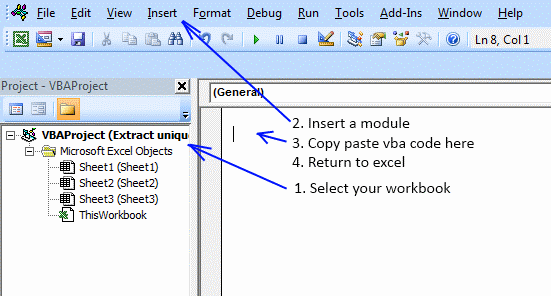Author: Oscar Cronquist Article last updated on January 12, 2021This article demonstrates two formulas that extract distinct values from a filtered Excel Table, one formula for Excel 365 subscribers and the other formula for earlier Excel versions.

Robert Jr asks:

Oscar,
I am using the VBA code & FilterUniqueSort array to generate unique lists that drive Selection Change AutoFilter on multiple colums. Is there a way to make the list only return the unique values that are visible in the filtered source data?

I see a similar answer above using just an array formula, but my source is too long for that to be practical. Any help would be greatly appreciated.

Robert Jr

### Update 1/10/2021 - dynamic array formula

Formula in cell B26:

=LET(x, Table2[First Name], UNIQUE(FILTER(x, SUBTOTAL(3, OFFSET(x, SEQUENCE(ROWS(x))-1, 0, 1))))

The formula above contains four new functions available to Excel 365 subscribers:

### Array formula

I modified a formula by Laurent Longre found here: Excel Experts E-letter from John Walkenbach's web site.

Array Formula in cell B26:

=INDEX(Table2[First Name], MATCH(0, IF(SUBTOTAL(3, OFFSET(Table2[First Name], MATCH(ROW(Table2[First Name]), ROW(Table2[First Name]))-1, 0, 1)), COUNTIF(\$C\$25:C25, Table2[First Name]),""), 0))

To enter an array formula, type the formula in a cell then press and hold CTRL + SHIFT simultaneously, now press Enter once. Release all keys.

The formula bar now shows the formula with a beginning and ending curly bracket telling you that you entered the formula successfully. Don't enter the curly brackets yourself.

### Explaining array formula in cell B26

#### Step 1 - Create array

This step is necessary in order to be able to use the SUBTOTAL function in the next step. The ROW function returns row numbers based on a cell reference.

The MATCH function converts the row number array to an array that starts with 1.

OFFSET(Table2[First Name], MATCH(ROW(Table2[First Name]), ROW(Table2[First Name]))-1, 0, 1)

becomes

OFFSET(Table2[First Name], MATCH({2; 3; 4; 5; 6; 7; 8; 9; 10; 11; 12; 13; 14; 15; 16; 17; 18; 19; 20; 21}, {2; 3; 4; 5; 6; 7; 8; 9; 10; 11; 12; 13; 14; 15; 16; 17; 18; 19; 20; 21})-1, 0, 1)

becomes

OFFSET(Table2[First Name], {1; 2; 3; 4; 5; 6; 7; 8; 9; 10; 11; 12; 13; 14; 15; 16; 17; 18; 19; 20}-1, 0, 1)

becomes

OFFSET(Table2[First Name], {0; 1; 2; 3; 4; 5; 6; 7; 8; 9; 10; 11; 12; 13; 14; 15; 16; 17; 18; 19}, 0, 1)

becomes

OFFSET({"Kaya"; "Fraser"; "Jui"; "Cyril"; "Spencer"; "Horace"; "Emmit"; "Lynna"; "Charley"; "Fraser"; "Mckinley"; "Tiara"; "Damien"; "Linnie"; "Jui"; "Fraser"; "Siena"; "Lynna"; "Kelton"; "Kaya"}, {0; 1; 2; 3; 4; 5; 6; 7; 8; 9; 10; 11; 12; 13; 14; 15; 16; 17; 18; 19}, 0, 1)

The OFFSET function creates an array of arrays that the SUBTOTAL function can process.

OFFSET({"Kaya"; "Fraser"; "Jui"; "Cyril"; "Spencer"; "Horace"; "Emmit"; "Lynna"; "Charley"; "Fraser"; "Mckinley"; "Tiara"; "Damien"; "Linnie"; "Jui"; "Fraser"; "Siena"; "Lynna"; "Kelton"; "Kaya"}, {0; 1; 2; 3; 4; 5; 6; 7; 8; 9; 10; 11; 12; 13; 14; 15; 16; 17; 18; 19}, 0, 1)

and returns

{ {"Kaya"}; {"Fraser"}; {"Jui"}; {"Cyril"}; {"Spencer"}; {"Horace"}; {"Emmit"}; {"Lynna"}; {"Charley"}; {"Fraser"}; {"Mckinley"}; {"Tiara"}; {"Damien"}; {"Linnie"}; {"Jui"}; {"Fraser"}; {"Siena"}; {"Lynna"}; {"Kelton"}; {"Kaya"} }

#### Step 2 - Which values are hidden?

The SUBTOTAL function will in this step count each array in the array as one and return 1 if the value is nonempty and is not visible. The first argument is 3 representing COUNTA function. It counts the number of cells that are not empty.

SUBTOTAL(3, OFFSET(Table2[First Name], MATCH(ROW(Table2[First Name]), ROW(Table2[First Name]))-1, 0, 1))

becomes

SUBTOTAL(3, { {"Kaya"}; {"Fraser"}; {"Jui"}; {"Cyril"}; {"Spencer"}; {"Horace"}; {"Emmit"}; {"Lynna"}; {"Charley"}; {"Fraser"}; {"Mckinley"}; {"Tiara"}; {"Damien"}; {"Linnie"}; {"Jui"}; {"Fraser"}; {"Siena"}; {"Lynna"}; {"Kelton"}; {"Kaya"} })

and returns

{1; 1; 1; 0; 0; 1; 0; 0; 0; 1; 0; 0; 0; 0; 1; 1; 0; 0; 1; 1}.

This array tells us that the three first values in Table2[First Name] are visible, however, the fourth value is hidden etc. 1 - visible, 0 (zero) - hidden.

#### Step 3 - Convert 1 to 0 (zero)

The IF function has three arguments, the first one must be a logical expression. If the expression evaluates to TRUE then one thing happens (argument 2) and if FALSE another thing happens (argument 3).

IF(SUBTOTAL(3, OFFSET(Table2[First Name], MATCH(ROW(Table2[First Name]), ROW(Table2[First Name]))-1, 0, 1)), COUNTIF(\$B\$25:B25, Table2[First Name]), "")

The COUNTIF function in this step will make sure that only unique distinct values are being returned, it contains an expanding cell reference that keeps track of previously displayed values.

IF(SUBTOTAL(3, OFFSET(Table2[First Name], MATCH(ROW(Table2[First Name]), ROW(Table2[First Name]))-1, 0, 1)), COUNTIF(\$B\$25:B25, Table2[First Name]), "")

becomes

IF({1; 1; 1; 0; 0; 1; 0; 0; 0; 1; 0; 0; 0; 0; 1; 1; 0; 0; 1; 1}, {0; 0; 0; 0; 0; 0; 0; 0; 0; 0; 0; 0; 0; 0; 0; 0; 0; 0; 0; 0}, "")

and returns

{0; 0; 0; ""; ""; 0; ""; ""; ""; 0; ""; ""; ""; ""; 0; 0; ""; ""; 0; 0}

#### Step 4 - Find position

The MATCH function returns a number representing the position of the first 0 (zero) in the array.

MATCH(0, IF(SUBTOTAL(3, OFFSET(Table2[First Name], MATCH(ROW(Table2[First Name]), ROW(Table2[First Name]))-1, 0, 1)), COUNTIF(\$C\$25:C25, Table2[First Name]),""), 0)

becomes

MATCH(0, {0; 0; 0; ""; ""; 0; ""; ""; ""; 0; ""; ""; ""; ""; 0; 0; ""; ""; 0; 0}, 0)

and returns 1.

#### Step 5 - Return value

The INDEX function returns a value based on cell reference and a row and column number.

INDEX(Table2[First Name], MATCH(0, IF(SUBTOTAL(3, OFFSET(Table2[First Name], MATCH(ROW(Table2[First Name]), ROW(Table2[First Name]))-1, 0, 1)), COUNTIF(\$C\$25:C25, Table2[First Name]),""), 0))

becomes

INDEX(Table2[First Name], 1)

and returns "Kaya" in cell B26.

### User defined FunctionUser defined function in cell range A26:A31:

=FilterUniqueSortTable(Table2[First Name])

This formula is also entered as an array formula.

#### User defined Function Syntax

FilterUniqueSortTable(rng)

#### Arguments

 Parameter Text rng Required. A cell reference to the range in the Excel defined Table you want to extract unique distinct values from.

#### VBA Code

```'Name custom function and parameters
Function FilterUniqueSortTable(rng As Range)

'Declare variables and data types
Dim ucoll As New Collection, Value As Variant, temp() As Variant
Dim iRows As Single, i As Single

'Redimension array variable temp in order to be able to expand the variable later on
ReDim temp(0)

'Enable error handling
On Error Resume Next

'Iterate thorugh each value in cell range
For Each Value In rng

'Check if cell is not empty and visible
If Len(Value) > 0 And Value.EntireRow.Hidden = False Then

'Add value to collection, this line will return an error if the value already exists in the collection
ucoll.Add Value, CStr(Value)
End If

'Continue with next value
Next Value

'Disable error handling
On Error GoTo 0

'Iterate through each value in collection
For Each Value In ucoll

'Save value to array variable temp
temp(UBound(temp)) = Value

'Add another container to array variable temp
ReDim Preserve temp(UBound(temp) + 1)

'Continue with next value
Next Value

'Remove last item from array variable temp
ReDim Preserve temp(UBound(temp) - 1)

'Count the number of rows the UDF is entered in by the user and save to variable iRows
iRows = Range(Application.Caller.Address).Rows.Count

'Use UDF SelectionSort to sort values from A to Z
SelectionSort temp

'Add items so the array variable temp has the same number of rows as the entered UDF and save blank values to items that contain nothing
For i = UBound(temp) To iRows
ReDim Preserve temp(UBound(temp) + 1)
temp(UBound(temp)) = ""
Next i

'Rearrange values in array variable temp and return them to worksheet
FilterUniqueSortTable = Application.Transpose(temp)
End Function

```

#### Where to copy code?Press Alt+F11 to open VB Editor.

1. Double click on your workbook in the Project Explorer.
2. Click "Insert" on the menu and then click on "Module".
3. Paste above code to code module.
4. Exit VB Editor and return to Excel.
Note, make sure you save your file with the file extension *.xlsm (Macro-enabled Workbook) in order to save the macro together with the workbook.

### Download Excel fileExtract-unique-distinct-values-from-a-filtered-tablev2.xlsm